Lecture 20 — Sorting¶

Overview¶

• Sorting is a fundamental operation
• Provides good practice in implementing and testing small functions
• Leads to a better understanding of algorithm efficiency
• Allows us to consider the fundamental notion of a merge of two sorted sequences.
• During testing, we will see an example of the important notion of passing functions as arguments.

Algorithms to Study¶

• Insertion sort
• Merge sort
• This is our primary focus.
• Python’s built in sort

Insertion Sort¶

• Idea:

• If we already have a sorted list and we want to insert a new value, we can shift values one location higher until we find the proper location for the new value
• Insert the new value
• Start with a just a list of length 1 and repeat until all values have been inserted
• Algorithm

for each index i in the list, starting at 1 do
Save the value stored at location i in variable x
Initialize j at location i-1
while j is non-negative and the location to insert x has not been found do
Shift the value at j up to j+1
Decrement j
Insert the value stored in x in location j+1
• Code (in-class exercise):

def ins_sort(v):

Steps to Testing¶

2. Insert print statements and/or view with debugger to see what it is actually doing.
3. Run on “test cases” that capture challenging conditions:
• Empty list
• Singleton list
• List of repeated values
• List where the largest value is at the beginning or the smallest value is at the end.

Rough Analysis of Time Required¶

• For any particular value of i in the outer for loop, there can be up to i-1 comparisons/shifts.
• When i==1 this is not much, but
• When i==N-1, this is a lot.
• Adding across the different values of i, this results in at most (roughly)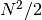comparisons.
• We write this as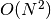because (informally) the number of comparisons done is proportional to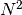.

Experimental Analysis¶

• The code test_sort.py posted on the Piazza site, and attached to the end of these notes, will be used to do timing experiments on all of the sorts we write.

• Makes use of the random module

• Includes three main functions:

• run_and_time
• run_and_time_python_sort
• generate_local_perm

We will discuss each of these in turn.

• The sorting functions themselves are functions in the module sort

• Notice that the sorting function is passed as an argument to run_and_time:

• First time that we have passed a function as an argument to another function!
• We will start with experiments to analyze selection sort (see textbook) and insertion sort.

Breaking the N-Squared Barrier¶

• The fundamental problem with both selection sort (discussed in the textbook, but not in these notes) and insertion sort:
• We need to do up to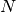comparisons by scanning through the list to find the proper location of the next value in the sorted.
• For insertion sort, we could use binary search to find the insertion location, but we would still have up toshifts of values.
• Do better than selection sort and insertion sort by using algorithms that don’t scan the entire list to assign one value.
• Examples
• Quick sort
• Heap sort
• Merge sort
• We’ll study merge sort, in part because it is the easiest of these to understand and in part because of the importance of the idea of a merge.

Merging Two Sorted Lists¶

• Given two lists that are each already sorted, our problem is to generate a new sorted list containing all of the items from both lists.

• For example,

L1 = [ 9, 12, 17, 25 ]
L2 = [ 3, 5, 11, 13, 16 ]

must be merged into a new list containing

[ 3, 5, 9, 11, 12, 13, 16, 17, 25 ]

• Idea:

• Since both lists are sorted, the first item in the new list must be the first item in one of the lists!
• If we “remove” the smallest item (3 in L1 in this case), the next item will again be the first non-copied item in one of the two lists!
• We repeat this process until one of the lists has no more items to copy.
• Then, copy the remainder of the other list to the back of our new list.
• We don’t actually remove the items from L1 or L2. Instead we keep an index to the next location of L1 and L2 that has not yet been copied.

• We’ll write the code in class, starting from here

def merge(L1, L2):
i1 = 0
i2 = 0
L = []

return L

• Exercises: These are based on the code we write in class.

1. Write the values of the index variables, i1 and i2, each time through the loop for lists L1 and L2 above.
2. What are the values of i1 and i2 when the loop terminates?

Merge Sort¶

• Key observation: all lists of length 1 are sorted

• Therefore, for a list of lengththat is to be sorted:

• Createlists of length 1 from the values in the list
• Start to merge these “singleton” lists in pairs to create longer, sorted lists.
• Repeat on pairs of longer lists in succession
• Requires

• Keeping a list of sorted sublists, initialized with each singleton list
• Rather than deleting the sorted sublists, just keep track of which we need to work on.
• Code (in class):

def merge_sort(v):
if len(v) <= 1:
return

Analysis of Merge Sort¶

• Check for correctness
• We’ll give an informal analysis explaining why there are only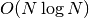comparisons.
• Experimental timings
• Can you think of ways to improve our implementation of the merge sort idea?

Final Comparison Across All Sorts¶

• Selection sort and insertion sort are dramatically slower than merge sort, which in turn is dramatically slower than Python’s built-in sort, a highly optimized, C language implementation of merge sort.

• Shows

• the difference betweensorting and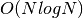sorting, and
• the difference between a straight-forward Python implementation and a careful, optimized implementation of the same algorithm.

Both of these are important!

• Final question: what happens when values are “almost” sorted?

• Experimentally, we can explore this using the generate_local_perm function in test_sort.py.
• Insertion sort becomes much faster, far outstripping selection sort. Why?

Practice Questions¶

1. For our insertion sort code, show the contents of the following list after each iteration of the outer for loop

v = [ 12, 4, 11, 2, 6, 18, 9 ]

While you can and should use the implementation to test your answers, you should start by manually generating the answers on your own.

2. Show the contents of the to_merge list at the end of the merge_sort implementation developed in class when it is called with

v = [ 17, 15, 29, 66, 31, 19, 9, 33 ].
3. Consider the following function

def extract( comp, v ):
x = v
for i in range(1,len(v)):
if comp(v[i],x):
x = v[i]
return x

Note that comp is a function that has been passed to extract.

1. Write a function called compare_lower(a,b) such that if L is a list then the call

extract(compare_lower,L)

returns the smallest value in L.

2. Write a function called compare_upper(a,b) such that if L is a list then the call

extract(compare_upper,L)

returns the largest value in L.

4. Write a version of merge that does all of the work inside the while loop and does not use the extend. This is a good test of your logic skills.

5. Based on your previous solution write a function to merge three sorted lists. This is an even greater challenge to your logic skills.

Note that when it comes to Test 3, you will not be required to have memorized the code of the sorting functions, but you should know the algorithms!